www.batmath.it

### Cramer's rule

Consider a linear system of n equations in n unknowns, written in compact form: Ax = b. If the matrix A is non singular the system has a unique solution that can easily be expressed by: x = A-1b. This means that writing the solution of such a system is much like the case of a linear equation in one unknown.

A consequence of this observation is an important theorem, known as Cramer's rule, that, though not much used in practice, is very important from a theoretical point of view because it reveals explicitly how the solution depends on the coefficients of the augmented matrix.

Given a linear system of n equations in n unknowns, call Δ the determinant of the matrix A of the system, Δi the determinant of the matrix formed by replacing the i-th column of the matrix A by the constants b1, b2, ...,bn. Then if ≠0 the unique solution of the system is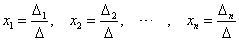.

#### Example

Solve the system Ax = b where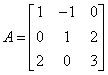and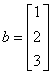. It's easy to find that det(A)=-1. By Cramer's rule we have: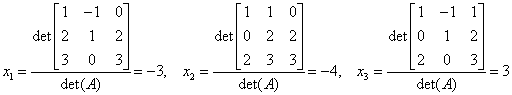.

We can easily check out the solution. We have: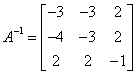. So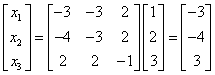first published on march 15 2002 - last updated on september 01 2003